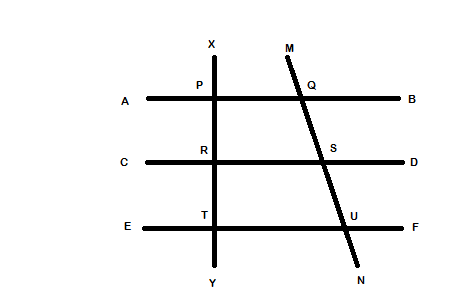## Geometry and Mensuration

Theory

● When two angles A and B are complementary, Sum of A and B = 90 degrees
● When two angles A and B are supplementary, Sum of A and B = 180
● When two lines intersect, opposite angles are equal. Adjacent angles are supplementary.
● When any number of lines intersect at a point, the sum of all angles formed = 360 degree

Theory● AB, CD, EF are three parallel lines
● XY and MN are known as transversals
● angle XPQ = angle PRS = angle RTU as corresponding angles are equal
● Interior angles on the side of the transversal are supplementary. i.e. angle PQS + angle QSR = 180 degrees
● Exterior angles on the same side of the transversal are supplementary. i.e. angle MQB + angle DSU = 180 degrees.
● Two transversals are cut by three parallel lines in the same ratio i.e. PR / RT = QS / SU

Theory

Triangles
● Sum of all angles in a triangle is 180 degrees
● An angle less than 90 degree is called an acute angle. An angle greater than 90 degrees is called an obtuse angle.
● A line joining the midpoint of a side with the opposite vertex is called a median.
● A perpendicular drawn from a vertex to the opposite side is called the altitude

Formula

Area of a triangle A
● A = $$\sqrt{s(s-a)(s-b)(s-c)}$$ where s = (a+b+c)/2.
● A = 1/2 * base * altitude
● A = 1/2 ab sinC
● Pythagoras theorem: In a right angled triangle ABC where angle B=90 degrees, $$AC^2 = AB^2 + BC^2$$

Formula

Surface area of different solids:
● Cube SA = $$6 * length^2$$
● Cylinder SA = $$2 \pi rh + 2 \pi r^2$$
● Cone SA = $$\pi r (l+r)$$ where r is radius of base and l is the slant height

Formula

Properties of a quadrilaterals (squares and rectangles)
● Area of a square with side $$l = l^2$$
● Perimeter of a square with side l = 4l
● Area of a rectangle with length l and breadth b = l*b
● Perimeter of a rectangle with length l and breadth b = 2(l+b)

Theory

Circle Properties
● The angle subtended by a diameter of circle on the circle = 90 degrees
● Length of the Circumference of a circle = $$2 \pi r$$
● Area of circle with radius r = $$\pi r^2$$

Formula

Volume of different solids:
● Cube $$V = length^3$$
● Cuboid V = length* base*height
● Cylinder $$V = \pi r^2 h$$
● Cone $$V = 1/3 * \pi r^2 *h$$

Solved Example

Side of a square is equal to the perimeter of a circle with diameter 14m. What will be the area of the square?
a: 1936 sq.m.
b: 1600 sq.m.
c: 1950 sq.m.
d: 1850 sq.m.

Explanation:

Perimeter of circle = $$2 \pi r$$ = 2 * 22/7 * 14/2 = 44m
Side of square = 44m
Area = $$44^2$$ = 1936 sq.m.

Solved Example

The side of a square is equal to the altitude of a triangle with area 200 sq.m. and base 5 m. What will be the area of square?
a: 6400 sq.m.
b: 1600 sq.m.
c: 3500 sq.m.
d: 3200 sq.m.

Explanation:

Area of triangle= 200 = 1/2*base*height
height = 200*2/5 = 80m
Therefore, side of square = 80m
Area of square = 6400 sq. m.